# RS Aggarwal Solutions for Class 7 Maths Chapter 6 Algebraic Expressions

RS Aggarwal Solutions for Class 7 Maths Chapter 6 – Algebraic Expressions are available here. Our expert tutors formulate these exercises to assist you with your exam preparation to attain good marks in Maths. Students who wish to score good marks in Maths diligently practise RS Aggarwal Solutions. Scoring high marks requires a good amount of practice on every topic. This book is one of the top materials when it comes to providing a question bank to practice.

The main aim is to help students understand and crack these problems. We at BYJU’S have prepared the RS Aggarwal Solutions for Class 7 Maths Chapter 6 wherein problems are solved step by step with detailed explanations. Download pdf of Class 7 Chapter 6 in their respective links.

## Download PDF of RS Aggarwal Solutions for Class 7 Maths Chapter 6 – Algebraic Expressions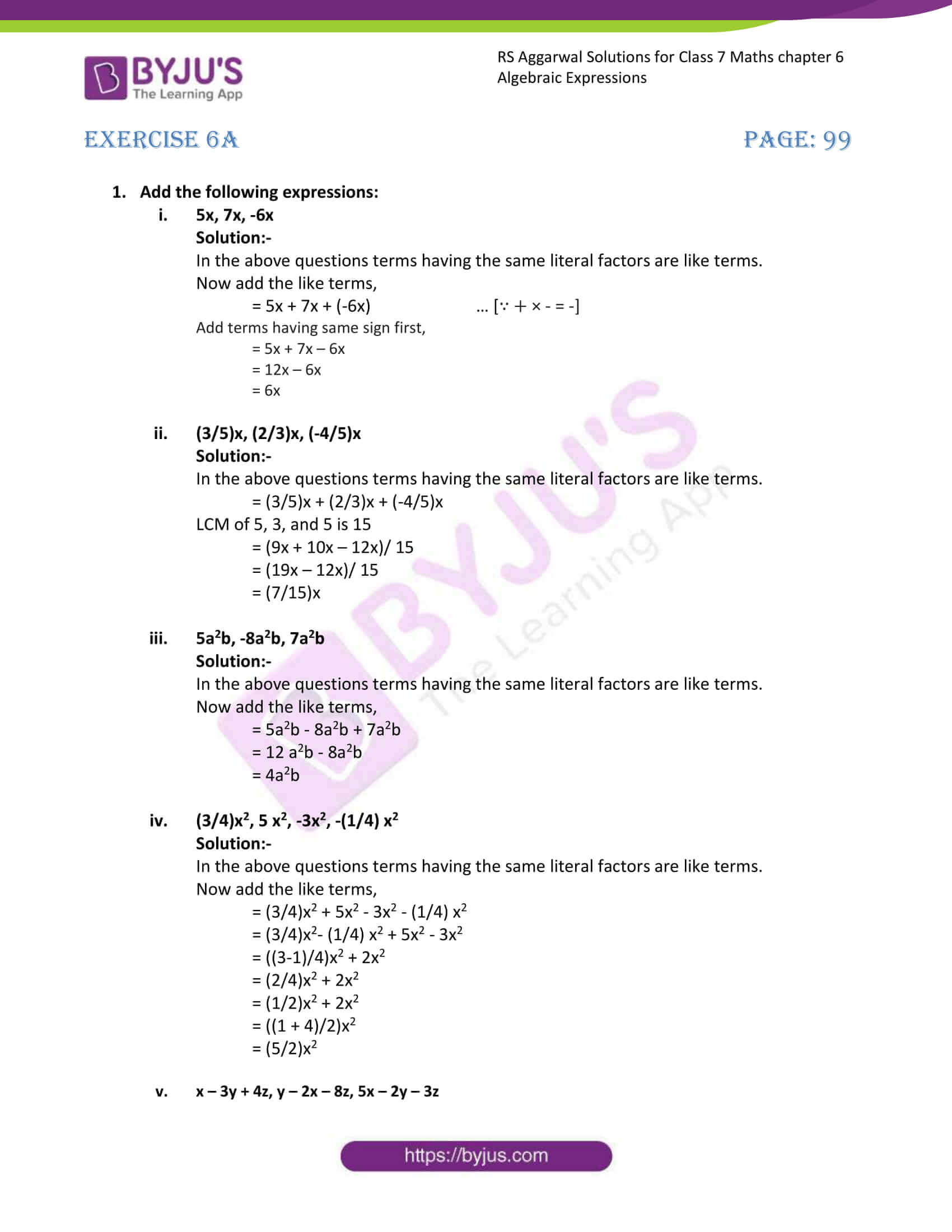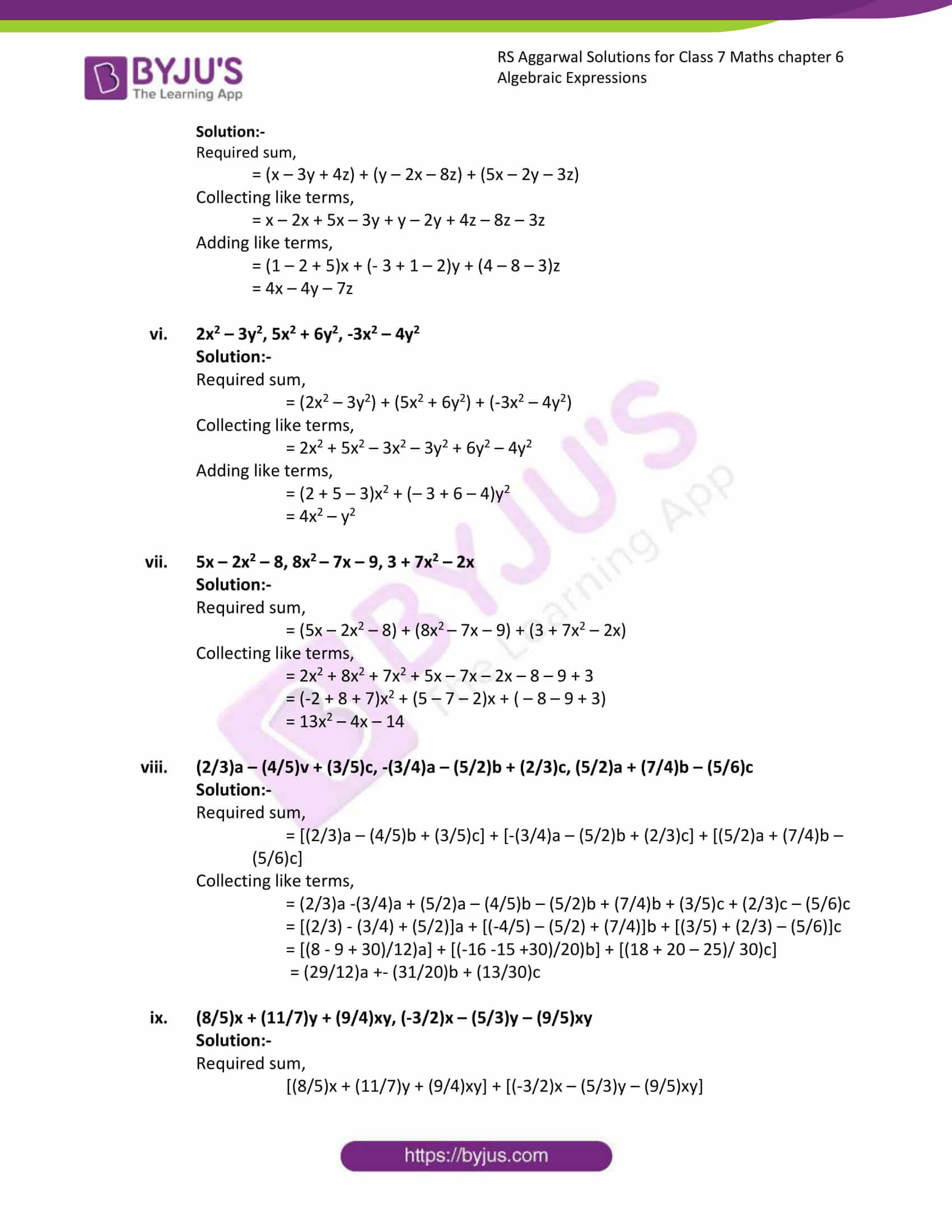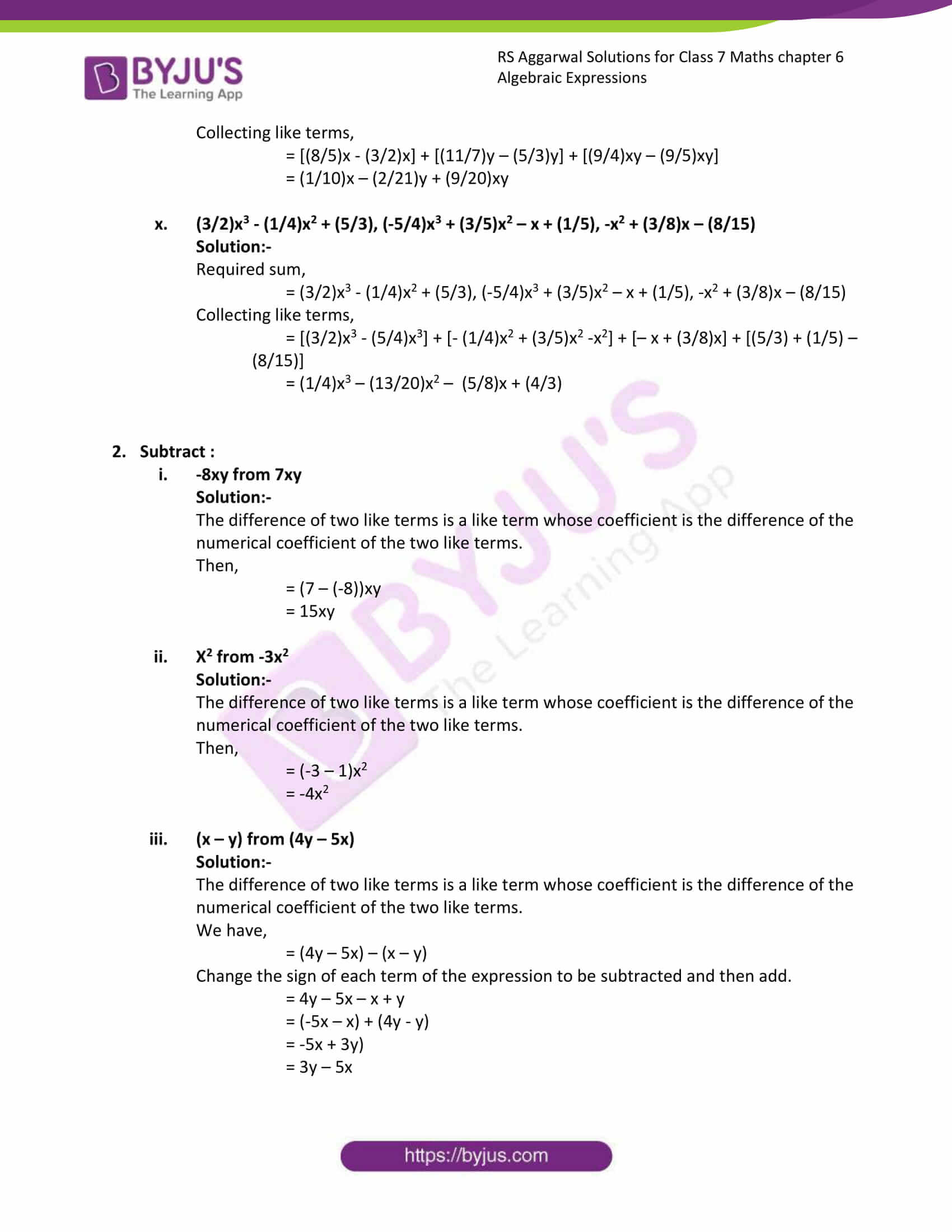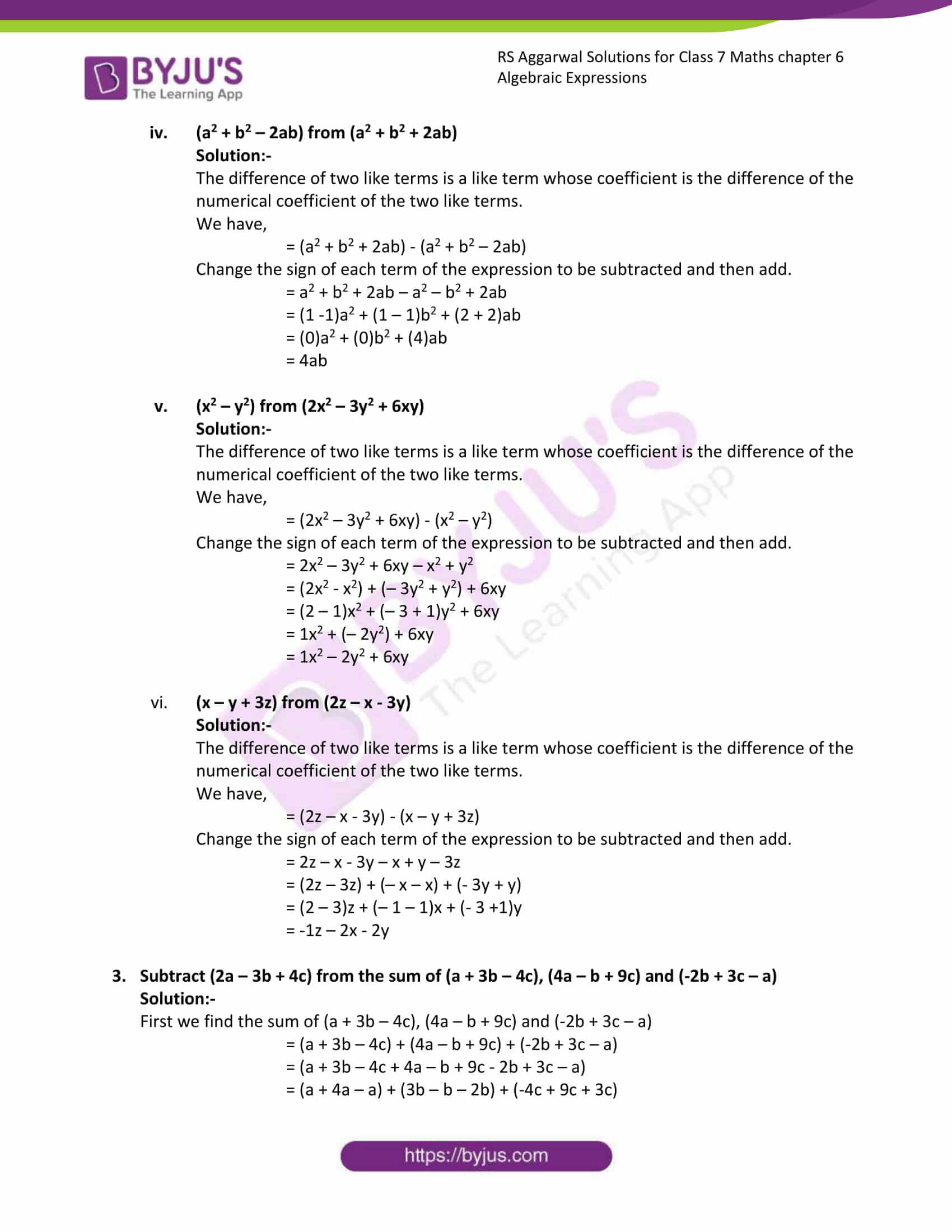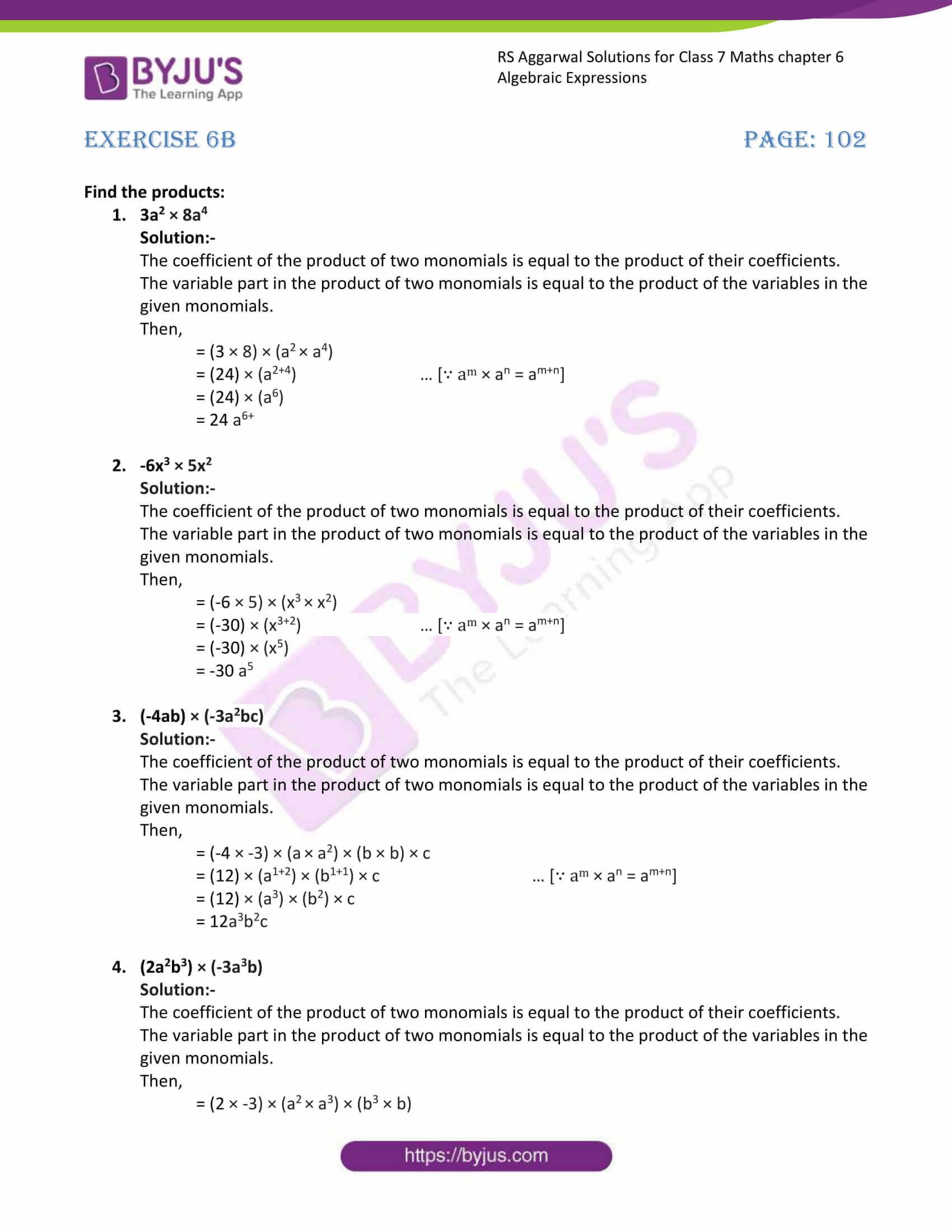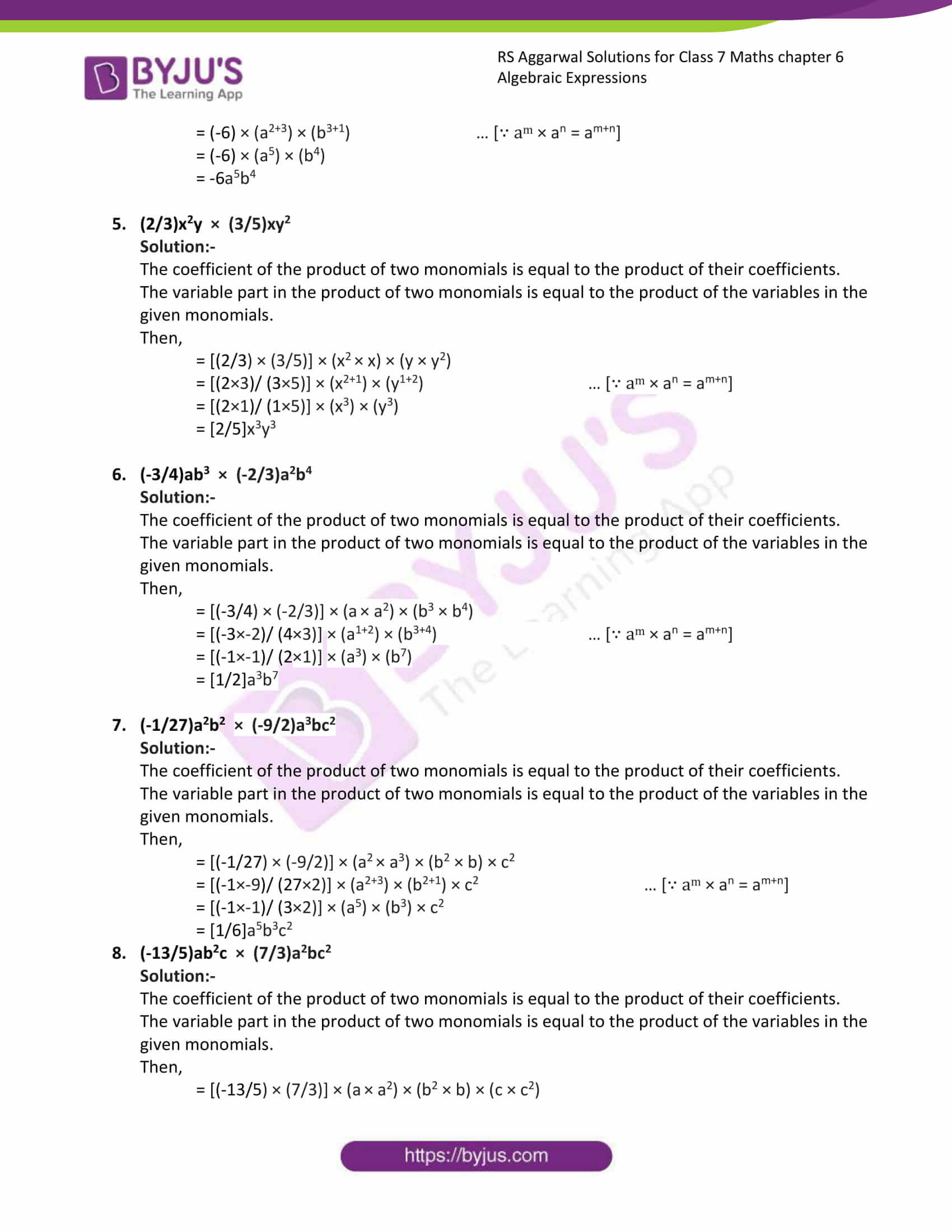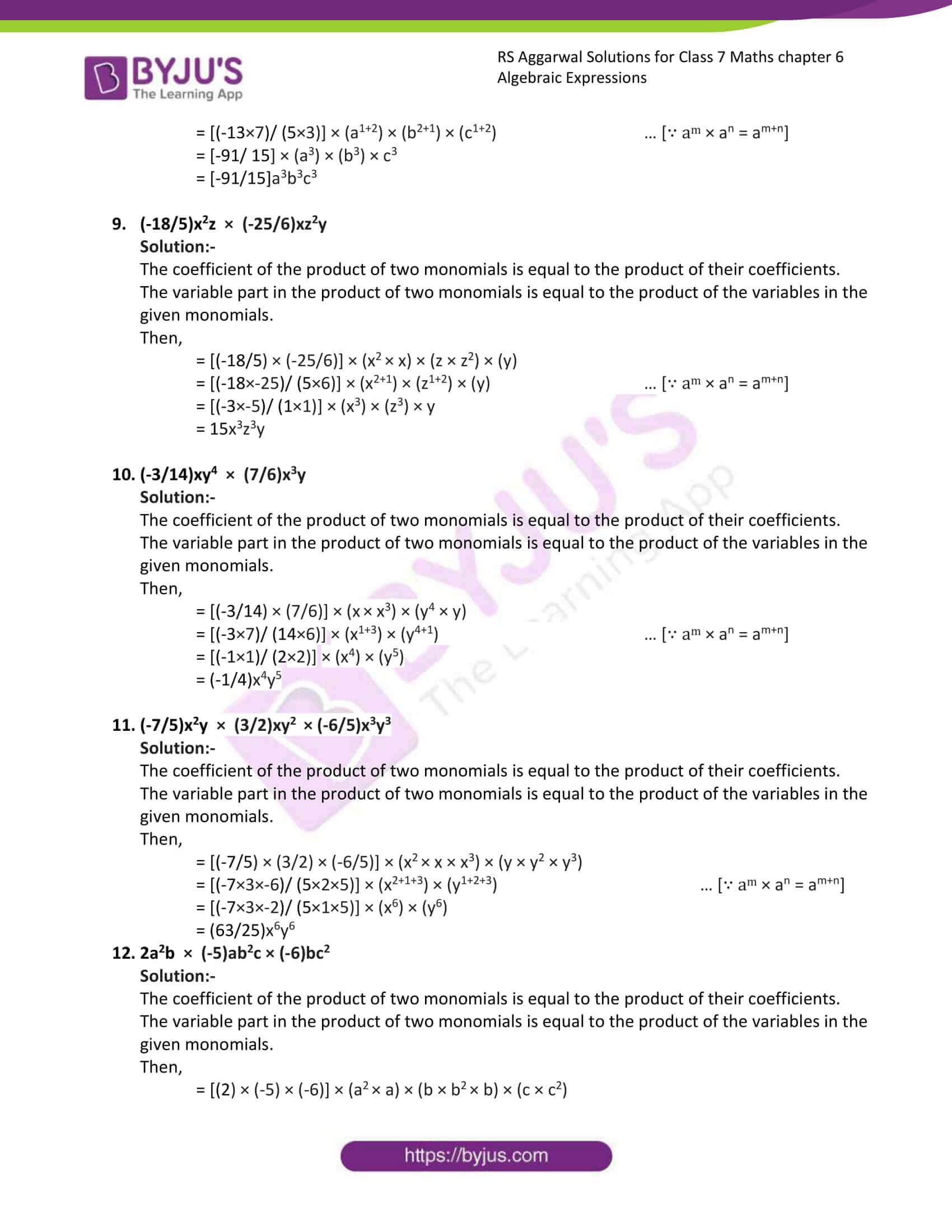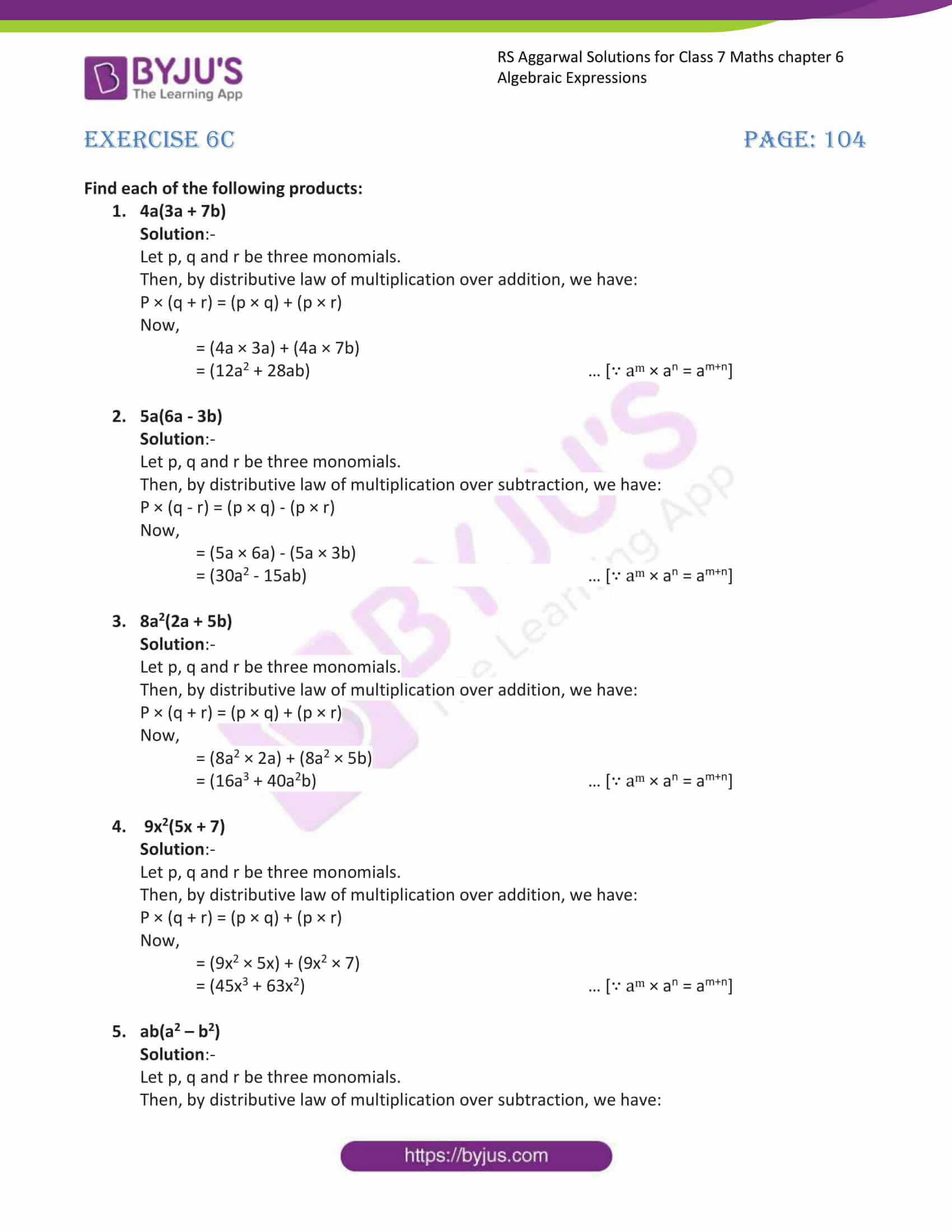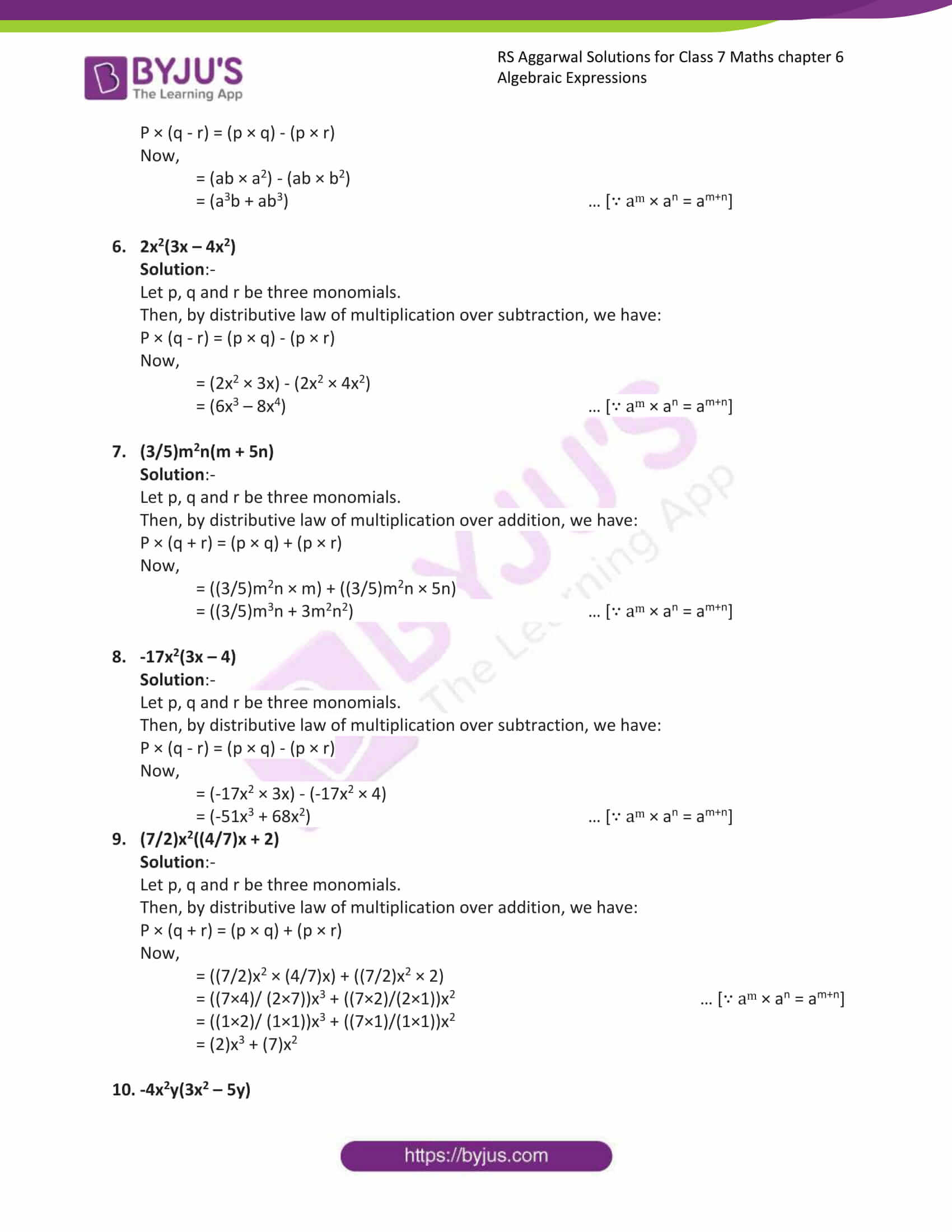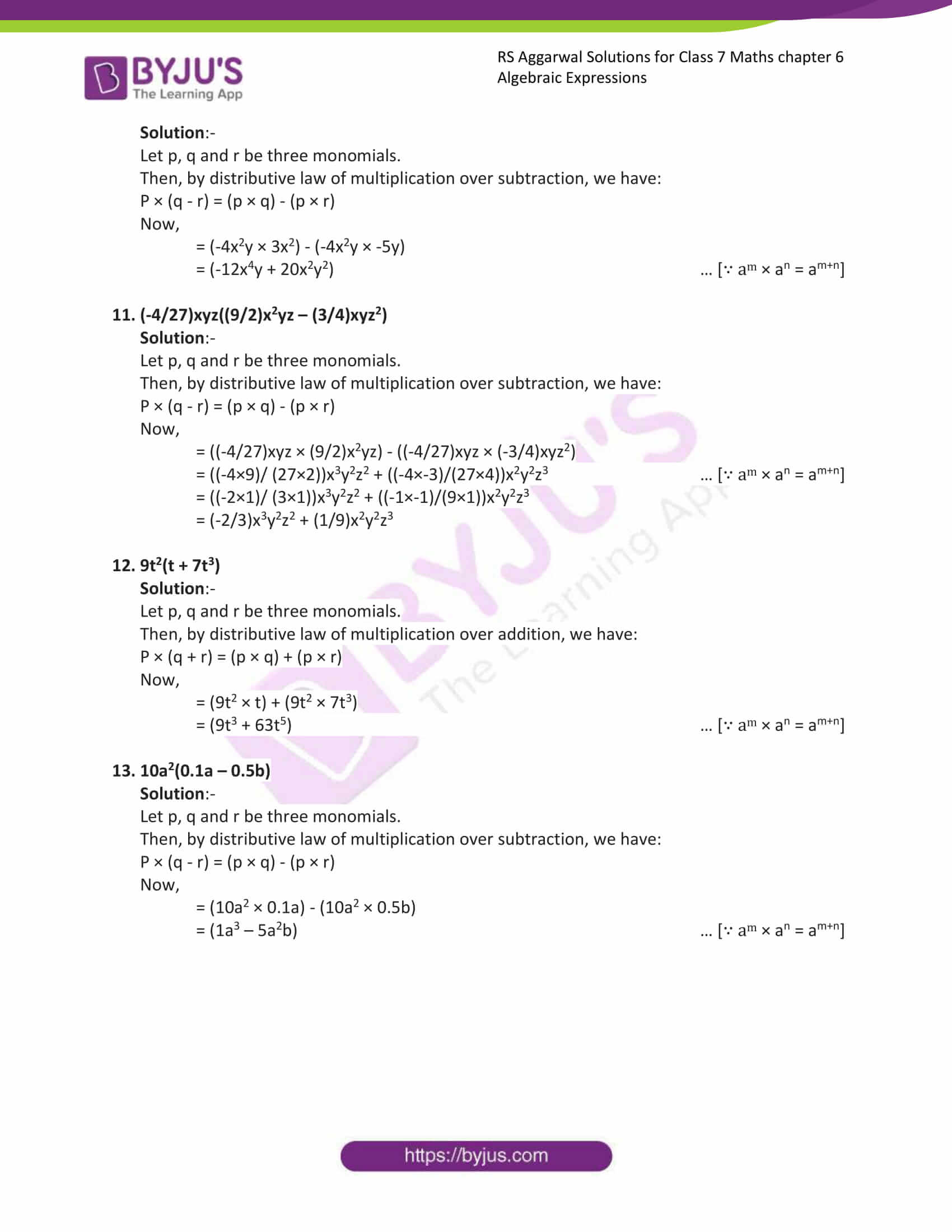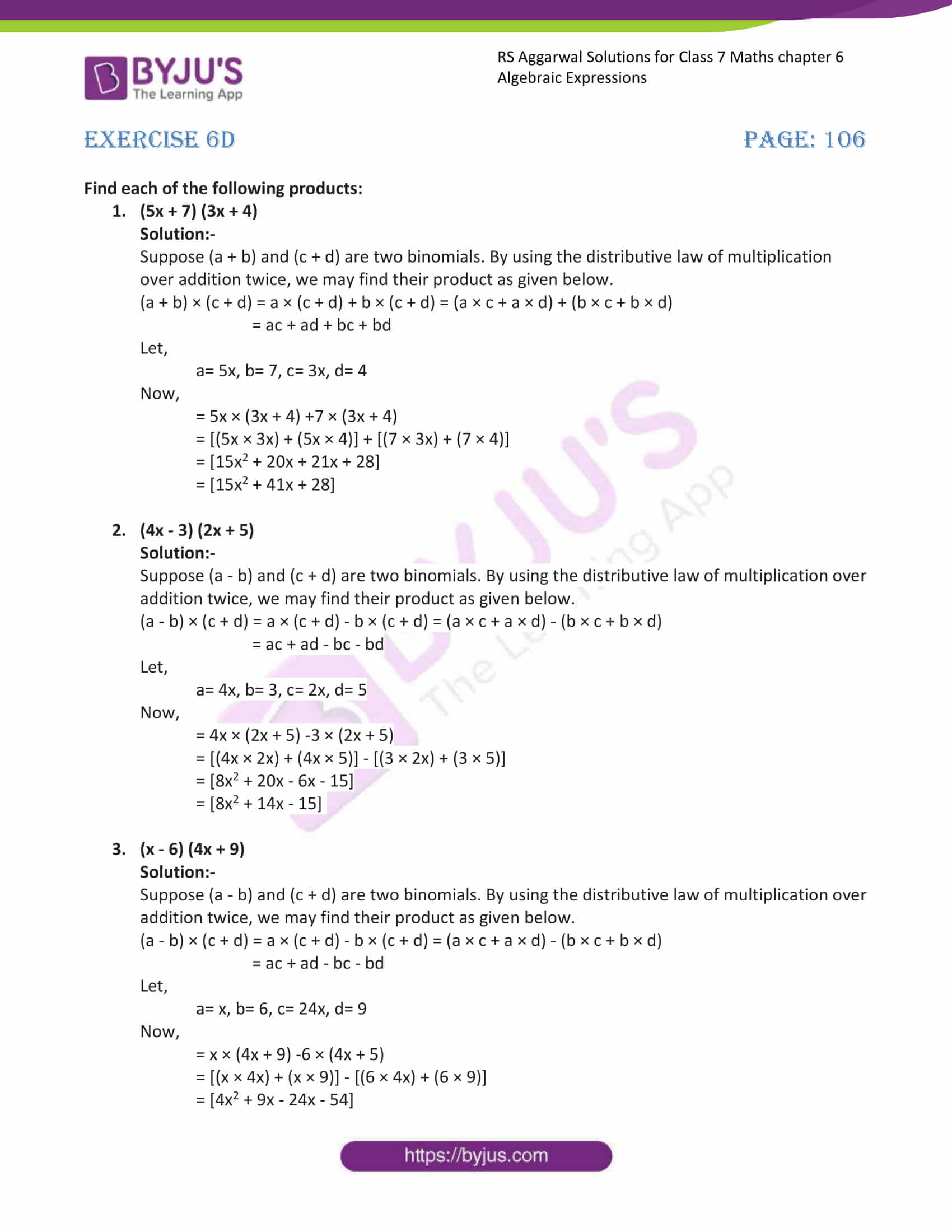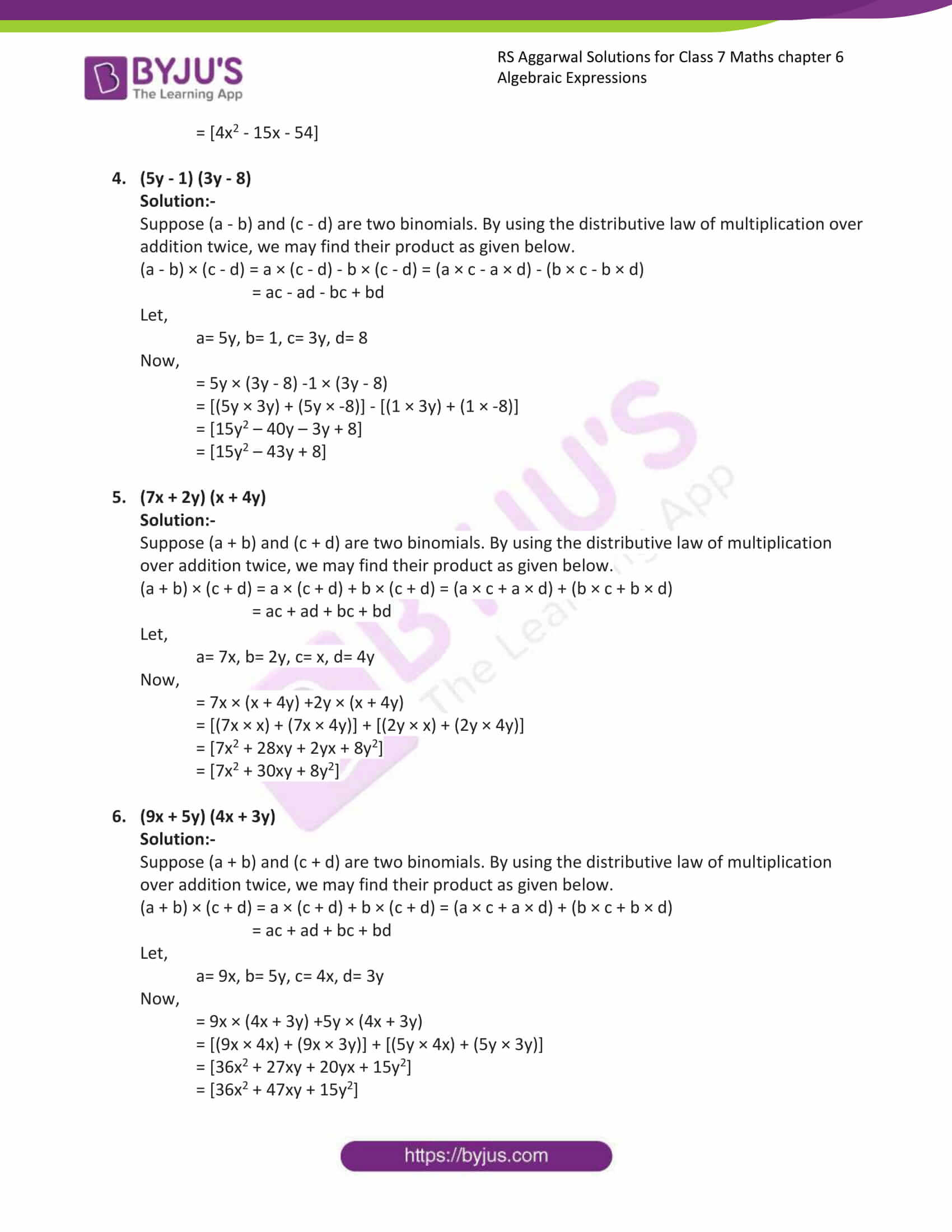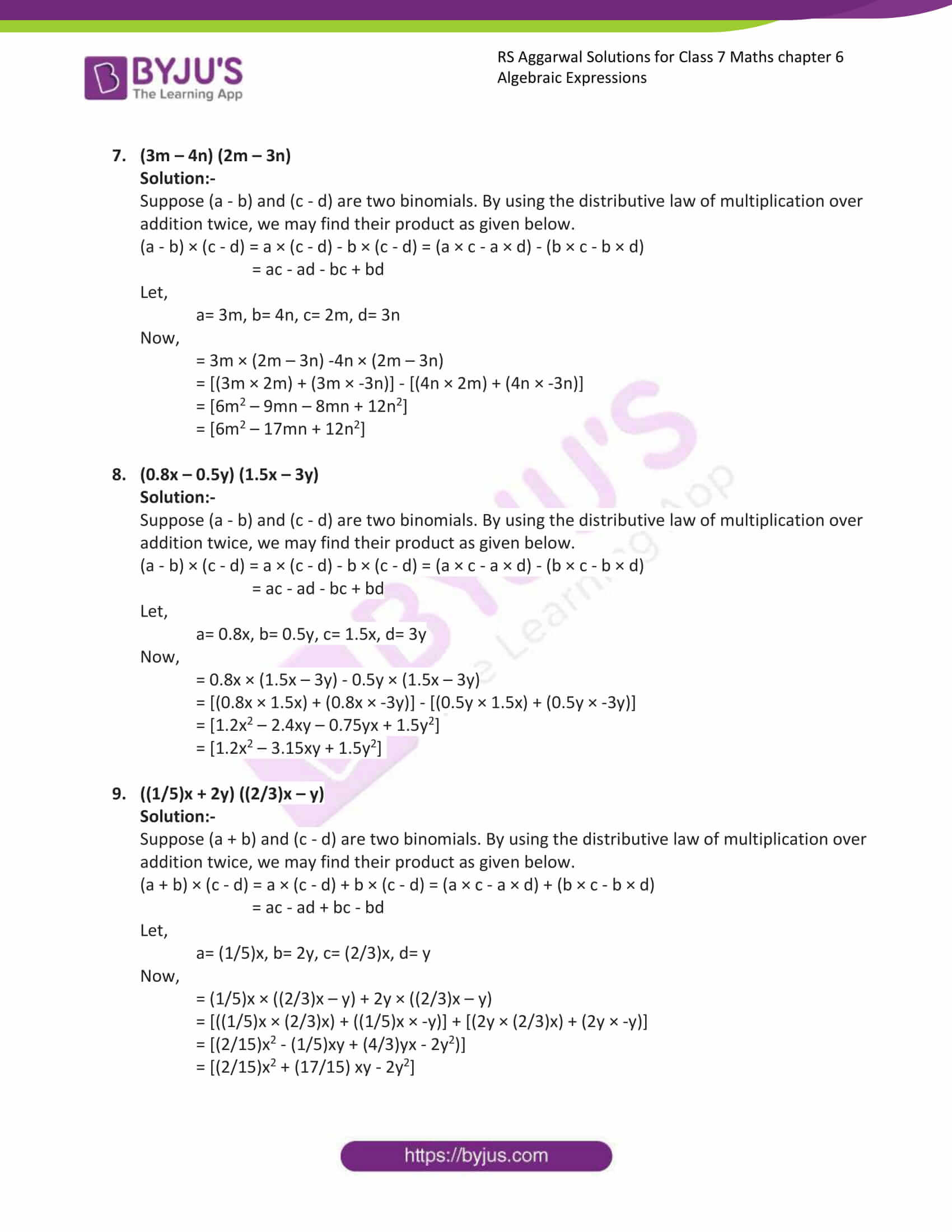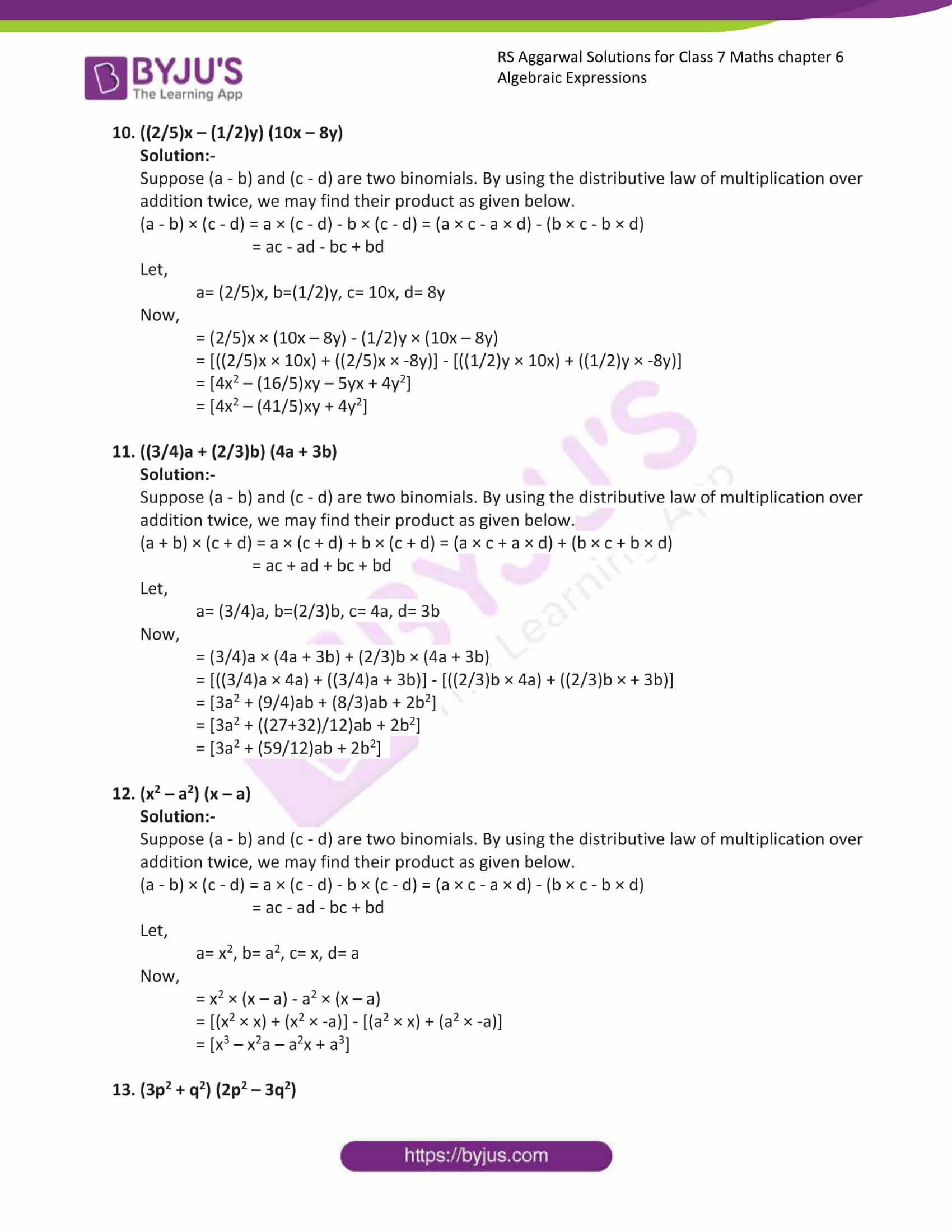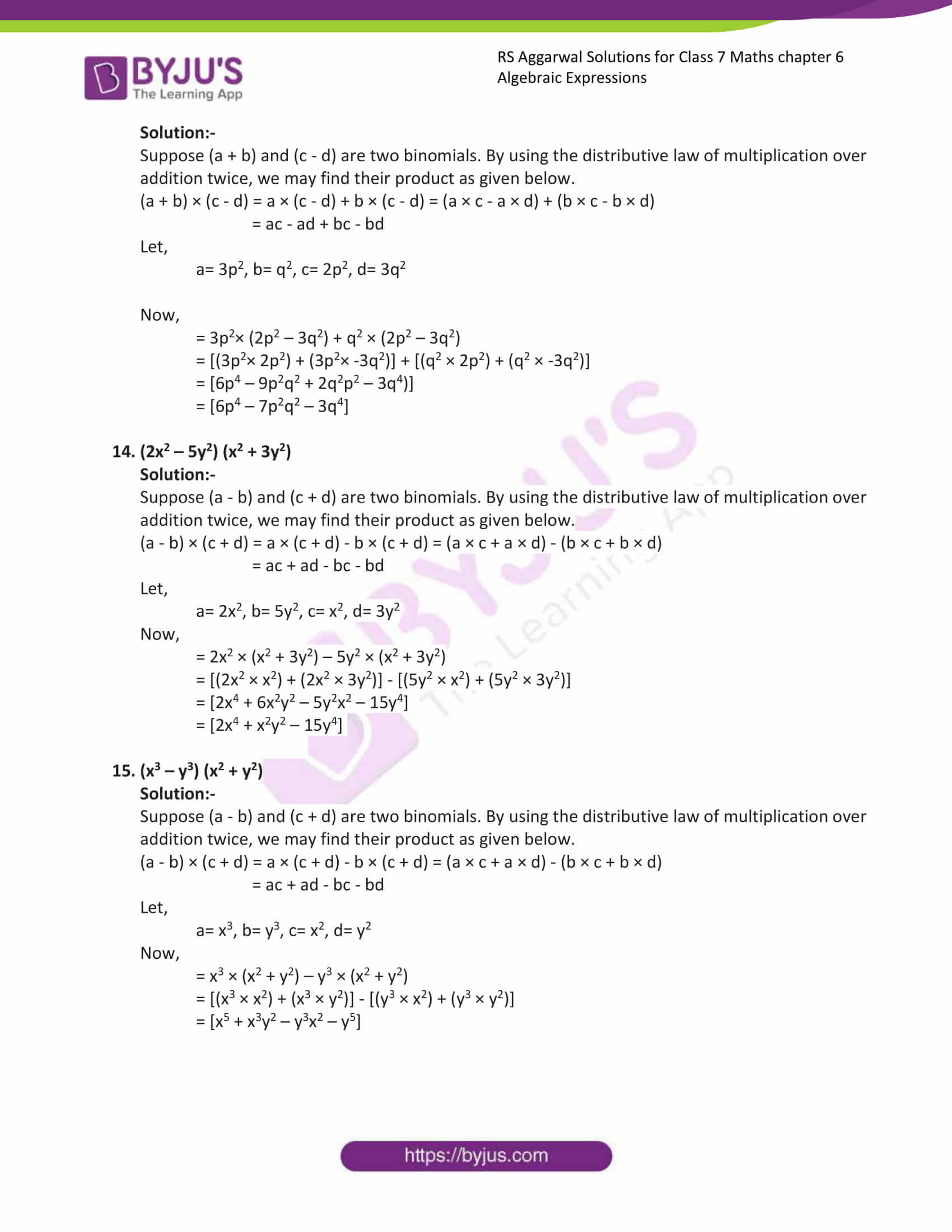Exercise 6A

Exercise 6B

Exercise 6C

Exercise 6D

## Exercise 6A

(i) 5x, 7x, -6x

Solution:-

In the above questions terms having the same literal factors are like terms.

= 5x + 7x + (-6x) … [∵ + × – = -]

Add terms having same sign first,

= 5x + 7x – 6x

= 12x – 6x

= 6x

(ii) (3/5)x, (2/3)x, (-4/5)x

Solution:-

In the above questions terms having the same literal factors are like terms.

= (3/5)x + (2/3)x + (-4/5)x

LCM of 5, 3, and 5 is 15

= (9x + 10x – 12x)/ 15

= (19x – 12x)/ 15

= (7/15)x

(iii) 5a2b, -8a2b, 7a2b

Solution:-

In the above questions terms having the same literal factors are like terms.

= 5a2b – 8a2b + 7a2b

= 12 a2b – 8a2b

= 4a2b

(iv) (3/4)x2, 5 x2, -3x2, -(1/4) x2

Solution:-

In the above questions terms having the same literal factors are like terms.

= (3/4)x2 + 5x2 – 3x2 – (1/4) x2

= (3/4)x2– (1/4) x2 + 5x2 – 3x2

= ((3-1)/4)x2 + 2x2

= (2/4)x2 + 2x2

= (1/2)x2 + 2x2

= ((1 + 4)/2)x2

= (5/2)x2

(v) x – 3y + 4z, y – 2x – 8z, 5x – 2y – 3z

Solution:-

Required sum,

= (x – 3y + 4z) + (y – 2x – 8z) + (5x – 2y – 3z)

Collecting like terms,

= x – 2x + 5x – 3y + y – 2y + 4z – 8z – 3z

= (1 – 2 + 5)x + (- 3 + 1 – 2)y + (4 – 8 – 3)z

= 4x – 4y – 7z

(vi) 2x2 – 3y2, 5x2 + 6y2, -3x2 – 4y2

Solution:-

Required sum,

= (2x2 – 3y2) + (5x2 + 6y2) + (-3x2 – 4y2)

Collecting like terms,

= 2x2 + 5x2 – 3x2 – 3y2 + 6y2 – 4y2

= (2 + 5 – 3)x2 + (– 3 + 6 – 4)y2

= 4x2 – y2

(vii) 5x – 2x2 – 8, 8x2 – 7x – 9, 3 + 7x2 – 2x

Solution:-

Required sum,

= (5x – 2x2 – 8) + (8x2 – 7x – 9) + (3 + 7x2 – 2x)

Collecting like terms,

= 2x2 + 8x2 + 7x2 + 5x – 7x – 2x – 8 – 9 + 3

= (-2 + 8 + 7)x2 + (5 – 7 – 2)x + ( – 8 – 9 + 3)

= 13x2 – 4x – 14

(viii) (2/3)a – (4/5)v + (3/5)c, -(3/4)a – (5/2)b + (2/3)c, (5/2)a + (7/4)b – (5/6)c

Solution:-

Required sum,

= [(2/3)a – (4/5)b + (3/5)c] + [-(3/4)a – (5/2)b + (2/3)c] + [(5/2)a + (7/4)b – (5/6)c]

Collecting like terms,

= (2/3)a -(3/4)a + (5/2)a – (4/5)b – (5/2)b + (7/4)b + (3/5)c + (2/3)c – (5/6)c

= [(2/3) – (3/4) + (5/2)]a + [(-4/5) – (5/2) + (7/4)]b + [(3/5) + (2/3) – (5/6)]c

= [(8 – 9 + 30)/12)a] + [(-16 -15 +30)/20)b] + [(18 + 20 – 25)/ 30)c]

= (29/12)a +- (31/20)b + (13/30)c

(ix) (8/5)x + (11/7)y + (9/4)xy, (-3/2)x – (5/3)y – (9/5)xy

Solution:-

Required sum,

[(8/5)x + (11/7)y + (9/4)xy] + [(-3/2)x – (5/3)y – (9/5)xy]

Collecting like terms,

= [(8/5)x – (3/2)x] + [(11/7)y – (5/3)y] + [(9/4)xy – (9/5)xy]

= (1/10)x – (2/21)y + (9/20)xy

(x) (3/2)x3 – (1/4)x2 + (5/3), (-5/4)x3 + (3/5)x2 – x + (1/5), -x2 + (3/8)x – (8/15)

Solution:-

Required sum,

= (3/2)x3 – (1/4)x2 + (5/3), (-5/4)x3 + (3/5)x2 – x + (1/5), -x2 + (3/8)x – (8/15)

Collecting like terms,

= [(3/2)x3 – (5/4)x3] + [- (1/4)x2 + (3/5)x2 -x2] + [– x + (3/8)x] + [(5/3) + (1/5) – (8/15)]

= (1/4)x3 – (13/20)x2 – (5/8)x + (4/3)

2. Subtract :

(i) -8xy from 7xy

Solution:-

The difference of two like terms is a like term whose coefficient is the difference of the numerical coefficient of the two like terms.

Then,

= (7 – (-8))xy

= 15xy

(ii) X2 from -3x2

Solution:-

The difference of two like terms is a like term whose coefficient is the difference of the numerical coefficient of the two like terms.

Then,

= (-3 – 1)x2

= -4x2

(iii) (x – y) from (4y – 5x)

Solution:-

The difference of two like terms is a like term whose coefficient is the difference of the numerical coefficient of the two like terms.

We have,

= (4y – 5x) – (x – y)

Change the sign of each term of the expression to be subtracted and then add.

= 4y – 5x – x + y

= (-5x – x) + (4y – y)

= -5x + 3y)

= 3y – 5x

(iv) (a2 + b2 – 2ab) from (a2 + b2 + 2ab)

Solution:-

The difference of two like terms is a like term whose coefficient is the difference of the numerical coefficient of the two like terms.

We have,

= (a2 + b2 + 2ab) – (a2 + b2 – 2ab)

Change the sign of each term of the expression to be subtracted and then add.

= a2 + b2 + 2ab – a2 – b2 + 2ab

= (1 -1)a2 + (1 – 1)b2 + (2 + 2)ab

= (0)a2 + (0)b2 + (4)ab

= 4ab

(v) (x2 – y2) from (2x2 – 3y2 + 6xy)

Solution:-

The difference of two like terms is a like term whose coefficient is the difference of the numerical coefficient of the two like terms.

We have,

= (2x2 – 3y2 + 6xy) – (x2 – y2)

Change the sign of each term of the expression to be subtracted and then add.

= 2x2 – 3y2 + 6xy – x2 + y2

= (2x2 – x2) + (– 3y2 + y2) + 6xy

= (2 – 1)x2 + (– 3 + 1)y2 + 6xy

= 1x2 + (– 2y2) + 6xy

= 1x2 – 2y2 + 6xy

(vi) (x – y + 3z) from (2z – x – 3y)

Solution:-

The difference of two like terms is a like term whose coefficient is the difference of the numerical coefficient of the two like terms.

We have,

= (2z – x – 3y) – (x – y + 3z)

Change the sign of each term of the expression to be subtracted and then add.

= 2z – x – 3y – x + y – 3z

= (2z – 3z) + (– x – x) + (- 3y + y)

= (2 – 3)z + (– 1 – 1)x + (- 3 +1)y

= -1z – 2x – 2y

3. Subtract (2a – 3b + 4c) from the sum of (a + 3b – 4c), (4a – b + 9c) and (-2b + 3c – a)

Solution:-

First we find the sum of (a + 3b – 4c), (4a – b + 9c) and (-2b + 3c – a)

= (a + 3b – 4c) + (4a – b + 9c) + (-2b + 3c – a)

= (a + 3b – 4c + 4a – b + 9c – 2b + 3c – a)

= (a + 4a – a) + (3b – b – 2b) + (-4c + 9c + 3c)

= (1 + 4 – 1)a + (3 – 1 – 2)b + (-4 + 9 + 3)c

= 4a + (0)b + 8c

=4a + 8c

Then,

Subtract (2a – 3b + 4c) from (4a + 8c)

= (4a + 8c) – (2a – 3b + 4c)

= 4a + 8c – 2a + 3b – 4c

= (4a – 2a) + (3b) + (8c – 4c)

= 2a + 3b + 4c

## Exercise 6B

Find the products:

1. 3a2 × 8a4

Solution:-

The coefficient of the product of two monomials is equal to the product of their coefficients.

The variable part in the product of two monomials is equal to the product of the variables in the given monomials.

Then,

= (3 × 8) × (a2 × a4)

= (24) × (a2+4) … [∵ am × an = am+n]

= (24) × (a6)

= 24 a6+

2. -6x3 × 5x2

Solution:-

The coefficient of the product of two monomials is equal to the product of their coefficients.

The variable part in the product of two monomials is equal to the product of the variables in the given monomials.

Then,

= (-6 × 5) × (x3 × x2)

= (-30) × (x3+2) … [∵ am × an = am+n]

= (-30) × (x5)

= -30 a5

3.(-4ab) × (-3a2bc)

Solution:-

The coefficient of the product of two monomials is equal to the product of their coefficients.

The variable part in the product of two monomials is equal to the product of the variables in the given monomials.

Then,

= (-4 × -3) × (a × a2) × (b × b) × c

= (12) × (a1+2) × (b1+1) × c … [∵ am × an = am+n]

= (12) × (a3) × (b2) × c

= 12a3b2c

4. (2a2b3) × (-3a3b)

Solution:-

The coefficient of the product of two monomials is equal to the product of their coefficients.

The variable part in the product of two monomials is equal to the product of the variables in the given monomials.

Then,

= (2 × -3) × (a2 × a3) × (b3 × b)

= (-6) × (a2+3) × (b3+1) … [∵ am × an = am+n]

= (-6) × (a5) × (b4)

= -6a5b4

5. (2/3)x2y × (3/5)xy2

Solution:-

The coefficient of the product of two monomials is equal to the product of their coefficients.

The variable part in the product of two monomials is equal to the product of the variables in the given monomials.

Then,

= [(2/3) × (3/5)] × (x2 × x) × (y × y2)

= [(2×3)/ (3×5)] × (x2+1) × (y1+2) … [∵ am × an = am+n]

= [(2×1)/ (1×5)] × (x3) × (y3)

= [2/5]x3y3

6. (-3/4)ab3 × (-2/3)a2b4

Solution:-

The coefficient of the product of two monomials is equal to the product of their coefficients.

The variable part in the product of two monomials is equal to the product of the variables in the given monomials.

Then,

= [(-3/4) × (-2/3)] × (a × a2) × (b3 × b4)

= [(-3×-2)/ (4×3)] × (a1+2) × (b3+4) … [∵ am × an = am+n]

= [(-1×-1)/ (2×1)] × (a3) × (b7)

= [1/2]a3b7

7. (-1/27)a2b2 × (-9/2)a3bc2

Solution:-

The coefficient of the product of two monomials is equal to the product of their coefficients.

The variable part in the product of two monomials is equal to the product of the variables in the given monomials.

Then,

= [(-1/27) × (-9/2)] × (a2 × a3) × (b2 × b) × c2

= [(-1×-9)/ (27×2)] × (a2+3) × (b2+1) × c2 … [∵ am × an = am+n]

= [(-1×-1)/ (3×2)] × (a5) × (b3) × c2

= [1/6]a5b3c2

8. (-13/5)ab2c × (7/3)a2bc2

Solution:-

The coefficient of the product of two monomials is equal to the product of their coefficients.

The variable part in the product of two monomials is equal to the product of the variables in the given monomials.

Then,

= [(-13/5) × (7/3)] × (a × a2) × (b2 × b) × (c × c2)

= [(-13×7)/ (5×3)] × (a1+2) × (b2+1) × (c1+2) … [∵ am × an = am+n]

= [-91/ 15] × (a3) × (b3) × c3

= [-91/15]a3b3c3

9. (-18/5)x2z × (-25/6)xz2y

Solution:-

The coefficient of the product of two monomials is equal to the product of their coefficients.

The variable part in the product of two monomials is equal to the product of the variables in the given monomials.

Then,

= [(-18/5) × (-25/6)] × (x2 × x) × (z × z2) × (y)

= [(-18×-25)/ (5×6)] × (x2+1) × (z1+2) × (y) … [∵ am × an = am+n]

= [(-3×-5)/ (1×1)] × (x3) × (z3) × y

= 15x3z3y

10. (-3/14)xy4 × (7/6)x3y

Solution:-

The coefficient of the product of two monomials is equal to the product of their coefficients.

The variable part in the product of two monomials is equal to the product of the variables in the given monomials.

Then,

= [(-3/14) × (7/6)] × (x × x3) × (y4 × y)

= [(-3×7)/ (14×6)] × (x1+3) × (y4+1) … [∵ am × an = am+n]

= [(-1×1)/ (2×2)] × (x4) × (y5)

= (-1/4)x4y5

11. (-7/5)x2y × (3/2)xy2 × (-6/5)x3y3

Solution:-

The coefficient of the product of two monomials is equal to the product of their coefficients.

The variable part in the product of two monomials is equal to the product of the variables in the given monomials.

Then,

= [(-7/5) × (3/2) × (-6/5)] × (x2 × x × x3) × (y × y2 × y3)

= [(-7×3×-6)/ (5×2×5)] × (x2+1+3) × (y1+2+3) … [∵ am × an = am+n]

= [(-7×3×-2)/ (5×1×5)] × (x6) × (y6)

= (63/25)x6y6

12. 2a2b × (-5)ab2c × (-6)bc2

Solution:-

The coefficient of the product of two monomials is equal to the product of their coefficients.

The variable part in the product of two monomials is equal to the product of the variables in the given monomials.

Then,

= [(2) × (-5) × (-6)] × (a2 × a) × (b × b2 × b) × (c × c2)

=  × (a2+1) × (b1+2+1) × (c1+2) … [∵ am × an = am+n]

=  × (a3) × (b4) × (c3)

= a3b4c3

## Exercise 6C

Find each of the following products:

1. 4a(3a + 7b)

Solution:-

Let p, q and r be three monomials.

Then, by distributive law of multiplication over addition, we have:

P × (q + r) = (p × q) + (p × r)

Now,

= (4a × 3a) + (4a × 7b)

= (12a2 + 28ab) … [∵ am × an = am+n]

2. 5a(6a – 3b)

Solution:-

Let p, q and r be three monomials.

Then, by distributive law of multiplication over subtraction, we have:

P × (q – r) = (p × q) – (p × r)

Now,

= (5a × 6a) – (5a × 3b)

= (30a2 – 15ab) … [∵ am × an = am+n]

3. 8a2(2a + 5b)

Solution:-

Let p, q and r be three monomials.

Then, by distributive law of multiplication over addition, we have:

P × (q + r) = (p × q) + (p × r)

Now,

= (8a2 × 2a) + (8a2 × 5b)

= (16a3 + 40a2b) … [∵ am × an = am+n]

4. 9x2(5x + 7)

Solution:-

Let p, q and r be three monomials.

Then, by distributive law of multiplication over addition, we have:

P × (q + r) = (p × q) + (p × r)

Now,

= (9x2 × 5x) + (9x2 × 7)

= (45x3 + 63x2) … [∵ am × an = am+n]

5. ab(a2 – b2)

Solution:-

Let p, q and r be three monomials.

Then, by distributive law of multiplication over subtraction, we have:

P × (q – r) = (p × q) – (p × r)

Now,

= (ab × a2) – (ab × b2)

= (a3b + ab3) … [∵ am × an = am+n]

6. 2x2(3x – 4x2)

Solution:-

Let p, q and r be three monomials.

Then, by distributive law of multiplication over subtraction, we have:

P × (q – r) = (p × q) – (p × r)

Now,

= (2x2 × 3x) – (2x2 × 4x2)

= (6x3 – 8x4) … [∵ am × an = am+n]

7.(3/5)m2n(m + 5n)

Solution:-

Let p, q and r be three monomials.

Then, by distributive law of multiplication over addition, we have:

P × (q + r) = (p × q) + (p × r)

Now,

= ((3/5)m2n × m) + ((3/5)m2n × 5n)

= ((3/5)m3n + 3m2n2) … [∵ am × an = am+n]

8. -17x2(3x – 4)

Solution:-

Let p, q and r be three monomials.

Then, by distributive law of multiplication over subtraction, we have:

P × (q – r) = (p × q) – (p × r)

Now,

= (-17x2 × 3x) – (-17x2 × 4)

= (-51x3 + 68x2) … [∵ am × an = am+n]

9. (7/2)x2((4/7)x + 2)

Solution:-

Let p, q and r be three monomials.

Then, by distributive law of multiplication over addition, we have:

P × (q + r) = (p × q) + (p × r)

Now,

= ((7/2)x2 × (4/7)x) + ((7/2)x2 × 2)

= ((7×4)/ (2×7))x3 + ((7×2)/(2×1))x2 … [∵ am × an = am+n]

= ((1×2)/ (1×1))x3 + ((7×1)/(1×1))x2

= (2)x3 + (7)x2

10. -4x2y(3x2 – 5y)

Solution:-

Let p, q and r be three monomials.

Then, by distributive law of multiplication over subtraction, we have:

P × (q – r) = (p × q) – (p × r)

Now,

= (-4x2y × 3x2) – (-4x2y × -5y)

= (-12x4y + 20x2y2) … [∵ am × an = am+n]

11. (-4/27)xyz((9/2)x2yz – (3/4)xyz2)

Solution:-

Let p, q and r be three monomials.

Then, by distributive law of multiplication over subtraction, we have:

P × (q – r) = (p × q) – (p × r)

Now,

= ((-4/27)xyz × (9/2)x2yz) – ((-4/27)xyz × (-3/4)xyz2)

= ((-4×9)/ (27×2))x3y2z2 + ((-4×-3)/(27×4))x2y2z3 … [∵ am × an = am+n]

= ((-2×1)/ (3×1))x3y2z2 + ((-1×-1)/(9×1))x2y2z3

= (-2/3)x3y2z2 + (1/9)x2y2z3

12. 9t2(t + 7t3)

Solution:-

Let p, q and r be three monomials.

Then, by distributive law of multiplication over addition, we have:

P × (q + r) = (p × q) + (p × r)

Now,

= (9t2 × t) + (9t2 × 7t3)

= (9t3 + 63t5) … [∵ am × an = am+n]

13. 10a2(0.1a – 0.5b)

Solution:-

Let p, q and r be three monomials.

Then, by distributive law of multiplication over subtraction, we have:

P × (q – r) = (p × q) – (p × r)

Now,

= (10a2 × 0.1a) – (10a2 × 0.5b)

= (1a3 – 5a2b) … [∵ am × an = am+n]

## Exercise 6D

Find each of the following products:

1. (5x + 7) (3x + 4)

Solution:-

Suppose (a + b) and (c + d) are two binomials. By using the distributive law of multiplication over addition twice, we may find their product as given below.

(a + b) × (c + d) = a × (c + d) + b × (c + d) = (a × c + a × d) + (b × c + b × d)

= ac + ad + bc + bd

Let,

a= 5x, b= 7, c= 3x, d= 4

Now,

= 5x × (3x + 4) +7 × (3x + 4)

= [(5x × 3x) + (5x × 4)] + [(7 × 3x) + (7 × 4)]

= [15x2 + 20x + 21x + 28]

= [15x2 + 41x + 28]

2. (4x – 3) (2x + 5)

Solution:-

Suppose (a – b) and (c + d) are two binomials. By using the distributive law of multiplication over addition twice, we may find their product as given below.

(a – b) × (c + d) = a × (c + d) – b × (c + d) = (a × c + a × d) – (b × c + b × d)

= ac + ad – bc – bd

Let,

a= 4x, b= 3, c= 2x, d= 5

Now,

= 4x × (2x + 5) -3 × (2x + 5)

= [(4x × 2x) + (4x × 5)] – [(3 × 2x) + (3 × 5)]

= [8x2 + 20x – 6x – 15]

= [8x2 + 14x – 15]

3. (x – 6) (4x + 9)

Solution:-

Suppose (a – b) and (c + d) are two binomials. By using the distributive law of multiplication over addition twice, we may find their product as given below.

(a – b) × (c + d) = a × (c + d) – b × (c + d) = (a × c + a × d) – (b × c + b × d)

= ac + ad – bc – bd

Let,

a= x, b= 6, c= 24x, d= 9

Now,

= x × (4x + 9) -6 × (4x + 5)

= [(x × 4x) + (x × 9)] – [(6 × 4x) + (6 × 9)]

= [4x2 + 9x – 24x – 54]

= [4x2 – 15x – 54]

4. (5y – 1) (3y – 8)

Solution:-

Suppose (a – b) and (c – d) are two binomials. By using the distributive law of multiplication over addition twice, we may find their product as given below.

(a – b) × (c – d) = a × (c – d) – b × (c – d) = (a × c – a × d) – (b × c – b × d)

= ac – ad – bc + bd

Let,

a= 5y, b= 1, c= 3y, d= 8

Now,

= 5y × (3y – 8) -1 × (3y – 8)

= [(5y × 3y) + (5y × -8)] – [(1 × 3y) + (1 × -8)]

= [15y2 – 40y – 3y + 8]

= [15y2 – 43y + 8]

5. (7x + 2y) (x + 4y)

Solution:-

Suppose (a + b) and (c + d) are two binomials. By using the distributive law of multiplication over addition twice, we may find their product as given below.

(a + b) × (c + d) = a × (c + d) + b × (c + d) = (a × c + a × d) + (b × c + b × d)

= ac + ad + bc + bd

Let,

a= 7x, b= 2y, c= x, d= 4y

Now,

= 7x × (x + 4y) +2y × (x + 4y)

= [(7x × x) + (7x × 4y)] + [(2y × x) + (2y × 4y)]

= [7x2 + 28xy + 2yx + 8y2]

= [7x2 + 30xy + 8y2]

6. (9x + 5y) (4x + 3y)

Solution:-

Suppose (a + b) and (c + d) are two binomials. By using the distributive law of multiplication over addition twice, we may find their product as given below.

(a + b) × (c + d) = a × (c + d) + b × (c + d) = (a × c + a × d) + (b × c + b × d)

= ac + ad + bc + bd

Let,

a= 9x, b= 5y, c= 4x, d= 3y

Now,

= 9x × (4x + 3y) +5y × (4x + 3y)

= [(9x × 4x) + (9x × 3y)] + [(5y × 4x) + (5y × 3y)]

= [36x2 + 27xy + 20yx + 15y2]

= [36x2 + 47xy + 15y2]

7. (3m – 4n) (2m – 3n)

Solution:-

Suppose (a – b) and (c – d) are two binomials. By using the distributive law of multiplication over addition twice, we may find their product as given below.

(a – b) × (c – d) = a × (c – d) – b × (c – d) = (a × c – a × d) – (b × c – b × d)

= ac – ad – bc + bd

Let,

a= 3m, b= 4n, c= 2m, d= 3n

Now,

= 3m × (2m – 3n) -4n × (2m – 3n)

= [(3m × 2m) + (3m × -3n)] – [(4n × 2m) + (4n × -3n)]

= [6m2 – 9mn – 8mn + 12n2]

= [6m2 – 17mn + 12n2]

8. (0.8x – 0.5y) (1.5x – 3y)

Solution:-

Suppose (a – b) and (c – d) are two binomials. By using the distributive law of multiplication over addition twice, we may find their product as given below.

(a – b) × (c – d) = a × (c – d) – b × (c – d) = (a × c – a × d) – (b × c – b × d)

= ac – ad – bc + bd

Let,

a= 0.8x, b= 0.5y, c= 1.5x, d= 3y

Now,

= 0.8x × (1.5x – 3y) – 0.5y × (1.5x – 3y)

= [(0.8x × 1.5x) + (0.8x × -3y)] – [(0.5y × 1.5x) + (0.5y × -3y)]

= [1.2x2 – 2.4xy – 0.75yx + 1.5y2]

= [1.2x2 – 3.15xy + 1.5y2]

9. ((1/5)x + 2y) ((2/3)x – y)

Solution:-

Suppose (a + b) and (c – d) are two binomials. By using the distributive law of multiplication over addition twice, we may find their product as given below.

(a + b) × (c – d) = a × (c – d) + b × (c – d) = (a × c – a × d) + (b × c – b × d)

= ac – ad + bc – bd

Let,

a= (1/5)x, b= 2y, c= (2/3)x, d= y

Now,

= (1/5)x × ((2/3)x – y) + 2y × ((2/3)x – y)

= [((1/5)x × (2/3)x) + ((1/5)x × -y)] + [(2y × (2/3)x) + (2y × -y)]

= [(2/15)x2 – (1/5)xy + (4/3)yx – 2y2)]

= [(2/15)x2 + (17/15) xy – 2y2]

10. ((2/5)x – (1/2)y) (10x – 8y)

Solution:-

Suppose (a – b) and (c – d) are two binomials. By using the distributive law of multiplication over addition twice, we may find their product as given below.

(a – b) × (c – d) = a × (c – d) – b × (c – d) = (a × c – a × d) – (b × c – b × d)

= ac – ad – bc + bd

Let,

a= (2/5)x, b=(1/2)y, c= 10x, d= 8y

Now,

= (2/5)x × (10x – 8y) – (1/2)y × (10x – 8y)

= [((2/5)x × 10x) + ((2/5)x × -8y)] – [((1/2)y × 10x) + ((1/2)y × -8y)]

= [4x2 – (16/5)xy – 5yx + 4y2]

= [4x2 – (41/5)xy + 4y2]

11. ((3/4)a + (2/3)b) (4a + 3b)

Solution:-

Suppose (a – b) and (c – d) are two binomials. By using the distributive law of multiplication over addition twice, we may find their product as given below.

(a + b) × (c + d) = a × (c + d) + b × (c + d) = (a × c + a × d) + (b × c + b × d)

= ac + ad + bc + bd

Let,

a= (3/4)a, b=(2/3)b, c= 4a, d= 3b

Now,

= (3/4)a × (4a + 3b) + (2/3)b × (4a + 3b)

= [((3/4)a × 4a) + ((3/4)a + 3b)] – [((2/3)b × 4a) + ((2/3)b × + 3b)]

= [3a2 + (9/4)ab + (8/3)ab + 2b2]

= [3a2 + ((27+32)/12)ab + 2b2]

= [3a2 + (59/12)ab + 2b2]

12. (x2 – a2) (x – a)

Solution:-

Suppose (a – b) and (c – d) are two binomials. By using the distributive law of multiplication over addition twice, we may find their product as given below.

(a – b) × (c – d) = a × (c – d) – b × (c – d) = (a × c – a × d) – (b × c – b × d)

= ac – ad – bc + bd

Let,

a= x2, b= a2, c= x, d= a

Now,

= x2 × (x – a) – a2 × (x – a)

= [(x2 × x) + (x2 × -a)] – [(a2 × x) + (a2 × -a)]

= [x3 – x2a – a2x + a3]

13. (3p2 + q2) (2p2 – 3q2)

Solution:-

Suppose (a + b) and (c – d) are two binomials. By using the distributive law of multiplication over addition twice, we may find their product as given below.

(a + b) × (c – d) = a × (c – d) + b × (c – d) = (a × c – a × d) + (b × c – b × d)

= ac – ad + bc – bd

Let,

a= 3p2, b= q2, c= 2p2, d= 3q2

Now,

= 3p2× (2p2 – 3q2) + q2 × (2p2 – 3q2)

= [(3p2× 2p2) + (3p2× -3q2)] + [(q2 × 2p2) + (q2 × -3q2)]

= [6p4 – 9p2q2 + 2q2p2 – 3q4)]

= [6p4 – 7p2q2 – 3q4]

14. (2x2 – 5y2) (x2 + 3y2)

Solution:-

Suppose (a – b) and (c + d) are two binomials. By using the distributive law of multiplication over addition twice, we may find their product as given below.

(a – b) × (c + d) = a × (c + d) – b × (c + d) = (a × c + a × d) – (b × c + b × d)

= ac + ad – bc – bd

Let,

a= 2x2, b= 5y2, c= x2, d= 3y2

Now,

= 2x2 × (x2 + 3y2) – 5y2 × (x2 + 3y2)

= [(2x2 × x2) + (2x2 × 3y2)] – [(5y2 × x2) + (5y2 × 3y2)]

= [2x4 + 6x2y2 – 5y2x2 – 15y4]

= [2x4 + x2y2 – 15y4]

15. (x3 – y3) (x2 + y2)

Solution:-

Suppose (a – b) and (c + d) are two binomials. By using the distributive law of multiplication over addition twice, we may find their product as given below.

(a – b) × (c + d) = a × (c + d) – b × (c + d) = (a × c + a × d) – (b × c + b × d)

= ac + ad – bc – bd

Let,

a= x3, b= y3, c= x2, d= y2

Now,

= x3 × (x2 + y2) – y3 × (x2 + y2)

= [(x3 × x2) + (x3 × y2)] – [(y3 × x2) + (y3 × y2)]

= [x5 + x3y2 – y3x2 – y5]

## RS Aggarwal Solutions for Class 7 Maths Chapter 6 – Algebraic Expressions

Chapter 6 – Algebraic Expressions contains 4 exercises and the RS Aggarwal Solutions available on this page provide solutions to the questions present in the exercises. Now, let us have a look at some of the concepts discussed in this chapter.

• Constants and Variables